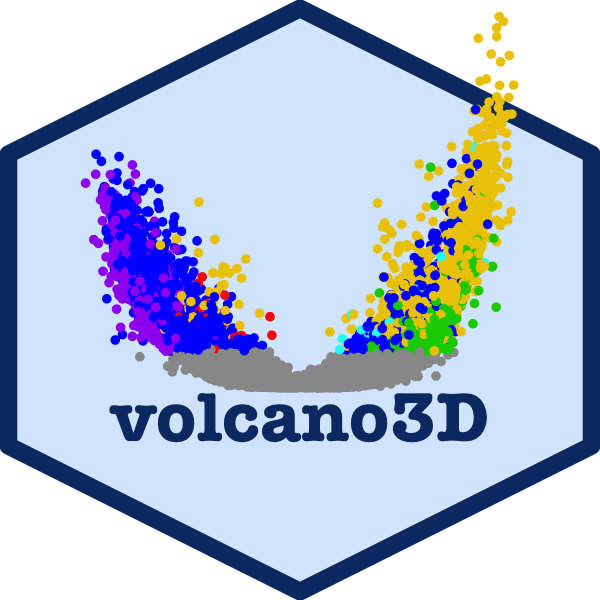# volcano3DThe volcano3D package provides a tool for analysis of three-class high dimensional data. It enables exploration of genes differentially expressed between three groups. Its main purpose is for the visualisation of differentially expressed genes in a three-dimensional volcano plot or three-way polar plot. These plots can be converted to interactive visualisations using plotly. The 3-way polar plots and 3d volcano plots can be applied to any data in which multiple attributes have been measured and their relative levels are being compared across three classes.

This vignette covers the basic features of the package using a small example data set. To explore more extensive examples and view the interactive radial and volcano plots, see the extended vignette which explores a case study from the PEAC rheumatoid arthritis trial (Pathobiology of Early Arthritis Cohort). The methodology has been published in Lewis, Myles J., et al. Molecular portraits of early rheumatoid arthritis identify clinical and treatment response phenotypes. Cell reports 28.9 (2019): 2455-2470. (DOI: 10.1016/j.celrep.2019.07.091) with an interactive, searchable web tool available at https://peac.hpc.qmul.ac.uk. This was creating as an R Shiny app and deployed to the web using a server.

There are also supplementary vignettes with further information on:

# Examples

This vignette uses a subset of the 500 genes from the PEAC dataset to explore the package functions. This can be loaded using:

``data("example_data")``

Which contains:

• `syn_example_rld` - the log transformed expression data

• `syn_example_meta` which contains sample information and divides the samples into 3 classes.

Samples in this cohort fall into three histological ‘pathotype’ groups:

``kable(table(syn_example_meta\$Pathotype), col.names = c("Pathotype", "Count"))``
Pathotype Count
Lymphoid 45
Myeloid 20
Fibroid 16

These will be used as the differential expression classes for the three-way analysis.

## Creating Polar Coordinates

First, the differential expression can be mapped to polar coordinates using the polar_coords function, which has inputs:

Variable Details

outcome

(required)
Vector containing three-level factor indicating which of the three classes each sample belongs to.

data

(required)
A dataframe or matrix containing data to be compared between the three classes (e.g. gene expression data). Note that variables are in columns, so gene expression data will need to be transposed. This is used to calculate z-score and fold change, so for gene expression count data it should be normalised such as log transformed or variance stabilised count transformation.

pvals

(optional)

the pvals matrix which contains the statistical significance of probes or attributes between classes. This contains:

• the first column is a group test such as one-way ANOVA or Kruskal-Wallis test.

• columns 2-4 contain p-values one for each comparison in the sequence A vs B, A vs C, B vs C, where A, B, C are the three levels in sequence in the outcome factor. For gene expression RNA-Seq count data, conduit functions using ‘limma voom’ or ‘DESeq’ pipelines to extract p-values for analysis are provided in functions `deseq_polar()` and `voom_polar()`. If p-values are not provided by the user, they can be calculated via the `polar_coords()` function.

(optional)
Matrix containing the adjusted p-values matching the pvals matrix.
pcutoff Cut-off for p-value significance
scheme Vector of colours starting with non-significant attributes
labs Optional character vector for labelling classes. Default `NULL` leads to abbreviated labels based on levels in `outcome` using `abbreviate()`. A vector of length 3 with custom abbreviated names for the outcome levels can be supplied. Otherwise a vector length 7 is expected, of the form “ns”, “B+”, “B+C+”, “C+”, “A+C+”, “A+”, “A+B+”, where “ns” means non-significant and A, B, C refer to levels 1, 2, 3 in `outcome`, and must be in the correct order.

This can be applied to the example data as below:

``````data("example_data")

syn_polar <- polar_coords(outcome = syn_example_meta\$Pathotype,
data = t(syn_example_rld))``````

This creates a ‘volc3d’ class object for downstream plotting.

## Gene expression pipeline

RNA-Sequencing gene expression count data can be compared for differentially expressed genes between 3 classes using 2 pipeline functions to allow statistical analysis by Bioconductor packages ‘DESeq2’ and ‘limma voom’ to quickly generate a polar plotting object of class ‘volc3d’ which can be plotted either as a 2d polar plot with 3 axes or as a 3d cylindrical plot with a 3d volcano plot.

### Method using DESeq2

This requires takes 2 `DESeqDataSet` objects and converts the results to a ‘volc3d’ class object for plotting. `object` is an object of class ‘DESeqDataSet’ with the full design formula. Note the function `DESeq` needs to have been previously run on this object. `objectLRT` is an object of class ‘DESeqDataSet’ with the reduced design formula. The function `DESeq` needs to have been run on this object with `DESeq` argument `test="LRT"`.

Note that in the DESeq2 design formula, the 3-class variable of interest should be first.

``````library(DESeq2)

# setup initial dataset from Tximport
dds <- DESeqDataSetFromTximport(txi = syn_txi,
design = ~ Pathotype + Batch + Gender)
# initial analysis run
dds_DE <- DESeq(dds)
# likelihood ratio test on 'Pathotype'
dds_LRT <- DESeq(dds, test = "LRT", reduced = ~ Batch + Gender, parallel = TRUE)

# create 'volc3d' class object for plotting
res <- deseq_polar(dds_DE, dds_LRT, "Pathotype")

# plot 3d volcano plot
volcano3D(res)``````

### Method using limma voom

The method for limma voom is faster and takes a design formula, metadata and raw count data. The Bioconductor packages ‘limma’ and ‘edgeR’ are used to analyse the data using the ‘voom’ method. The results are converted to a ‘volc3d’ object ready for plotting a 3d volcano plot or 3-way polar plot.

Note the design formula must be of the form `~ 0 + outcome + ...`. The 3-class outcome variable must be the first variable after the ‘0’, and this variable must be a factor with exactly 3 levels.

``````library(limma)
library(edgeR)

syn_tpm <- syn_txi\$counts  # raw counts

resl <- voom_polar(~ 0 + Pathotype + Batch + Gender, syn_metadata, syn_tpm)

volcano3D(resl)``````

The differential expression can now be visualised on an interactive radial plot using `radial_plotly`.
``radial_plotly(syn_polar) ``
A very similar looking static ggplot image can be created using `radial_ggplot`:
``````radial_ggplot(syn_polar,